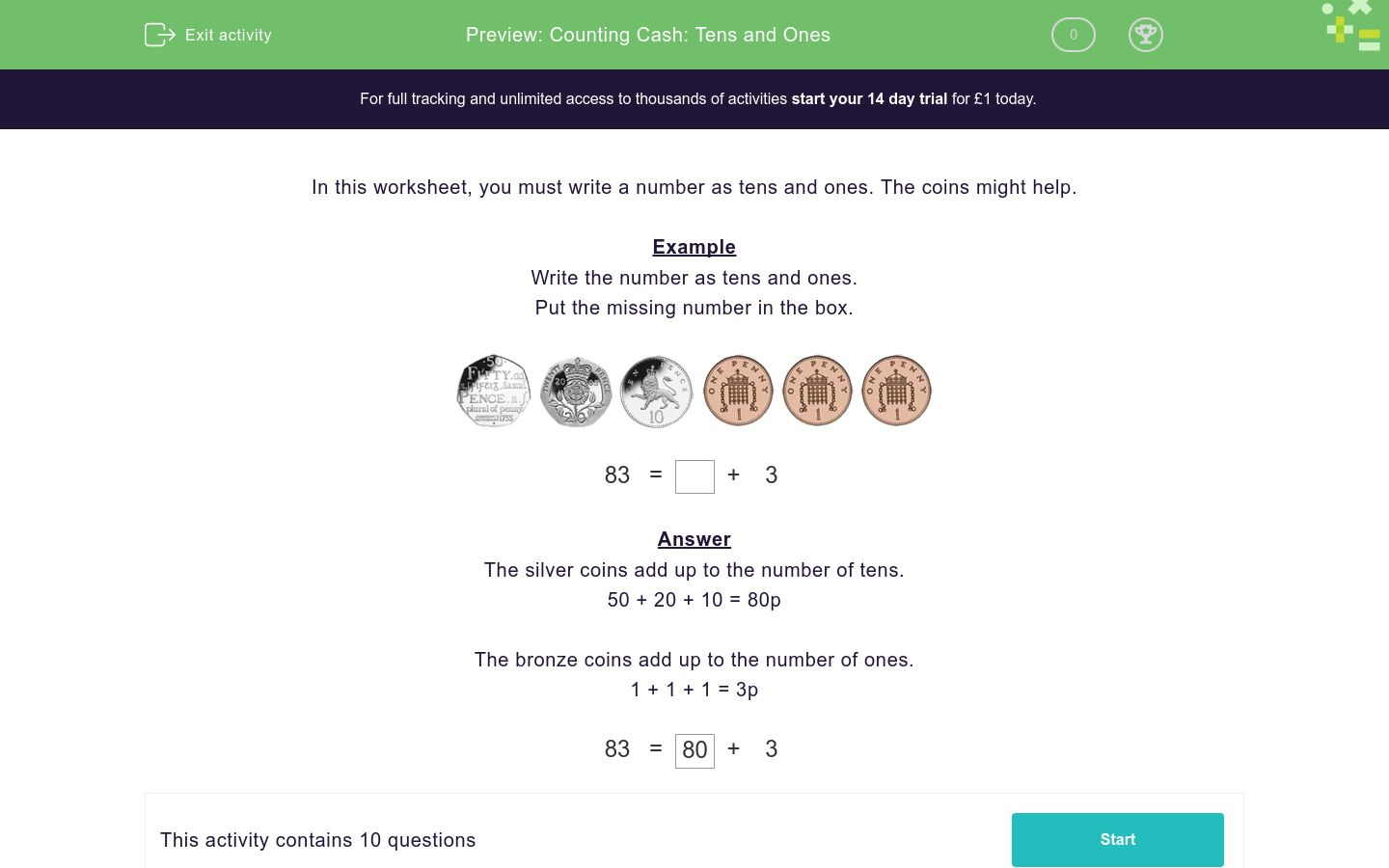# Counting Cash: Tens and Ones

In this worksheet, students use coins of different denominations to help them to count using tens and ones.Key stage:  KS 1

Curriculum topic:   Measurement

Curriculum subtopic:   Use Combinations of Coins

Difficulty level:#### Worksheet Overview

In this worksheet, you must write a number as tens and ones. The coins might help.

Example

Write the number as tens and ones.

Put the missing number in the box.83 = + 3

The silver coins add up to the number of tens.

50 + 20 + 10 = 80p

The bronze coins add up to the number of ones.

1 + 1 + 1 = 3p

 83 = 80 + 3

### What is EdPlace?

We're your National Curriculum aligned online education content provider helping each child succeed in English, maths and science from year 1 to GCSE. With an EdPlace account you’ll be able to track and measure progress, helping each child achieve their best. We build confidence and attainment by personalising each child’s learning at a level that suits them.

Get started